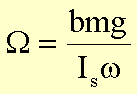# How does the gyroscopic precession work

Precession of a top

A symmetrical top is a rigid body that rotates around a fixed point O and has a dynamic axis of symmetry (z'-axis) that goes through its center of gravity. If the point O coincides with the center of gravity of the top, the top is free of forces in the gravitational field. Otherwise one speaks of a heavy top. We consider a top under the influence of an external force F, which acts on the starting point A. In the case of gravity, point A is identical to the center of gravity of the top. The gyro rotates around its axis of symmetry with the frequency w. This is the moment of inertia when rotating around this axis I.z ’= I.S.so is the angular momentum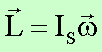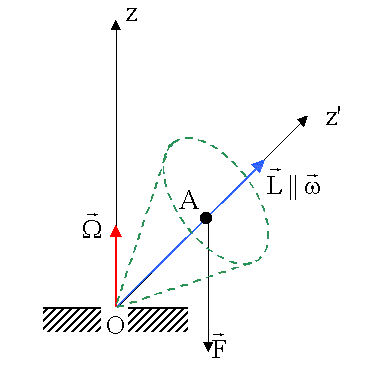The force F generates a torque D, which is perpendicular to L (in the drawing above, perpendicular to the plane of the drawing). This torque generates a change in angular momentum dLy perpendicular to L: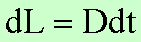Under the influence of the change in angular momentum dL an additional rotation of the gyro around the z-axis with the angular velocity W. This movement is called precession. With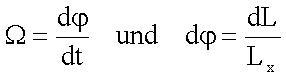you get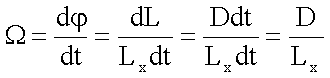or.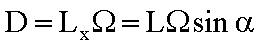This generally follows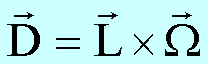We discuss the relationship between angular momentum, torque and precession frequency using the precession motion of a wheel as an example: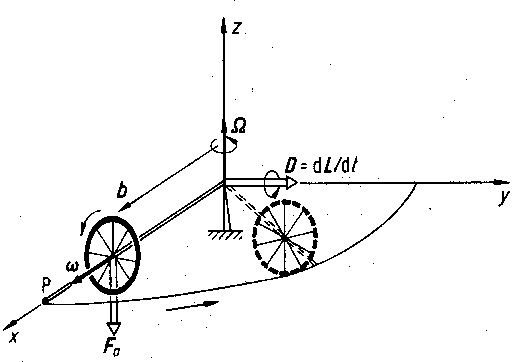The wheel rotating with the frequency w about its axis of symmetry is supported on the axis outside of its center of gravity. The torque generated by its own weight mg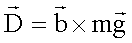leads to a precession with the frequency W about the z-axis. Because of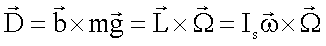as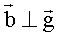and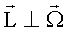it follows for the amount of W: